# Class 10 – Area Related To Circles – Previous Years Questions

Area Related To Circles – Previous Years Questions

1. In the given figure, O is the centre of a circle such that diameter AB = 13 cm and AC = 12 cm. BC is joined. Find the area of the shaded region. (Take π = 3.14)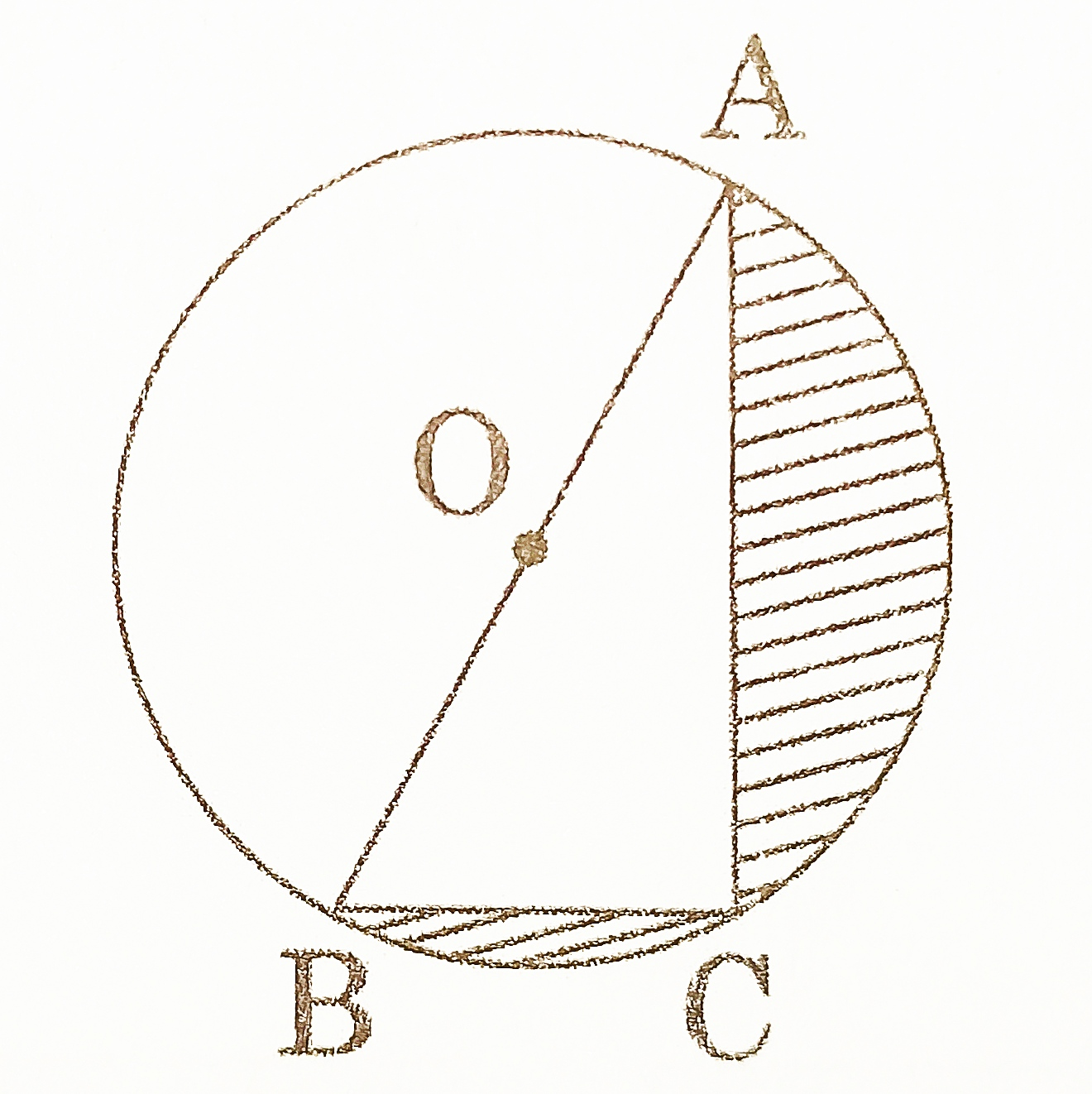[CBSE 2016] [3 Marks]
2. In the given figure, find the area of the shaded region, enclosed between two concentric circles of radii 7 cm and 14 cm where ∠AOC = 40°. (Use π = 22/7)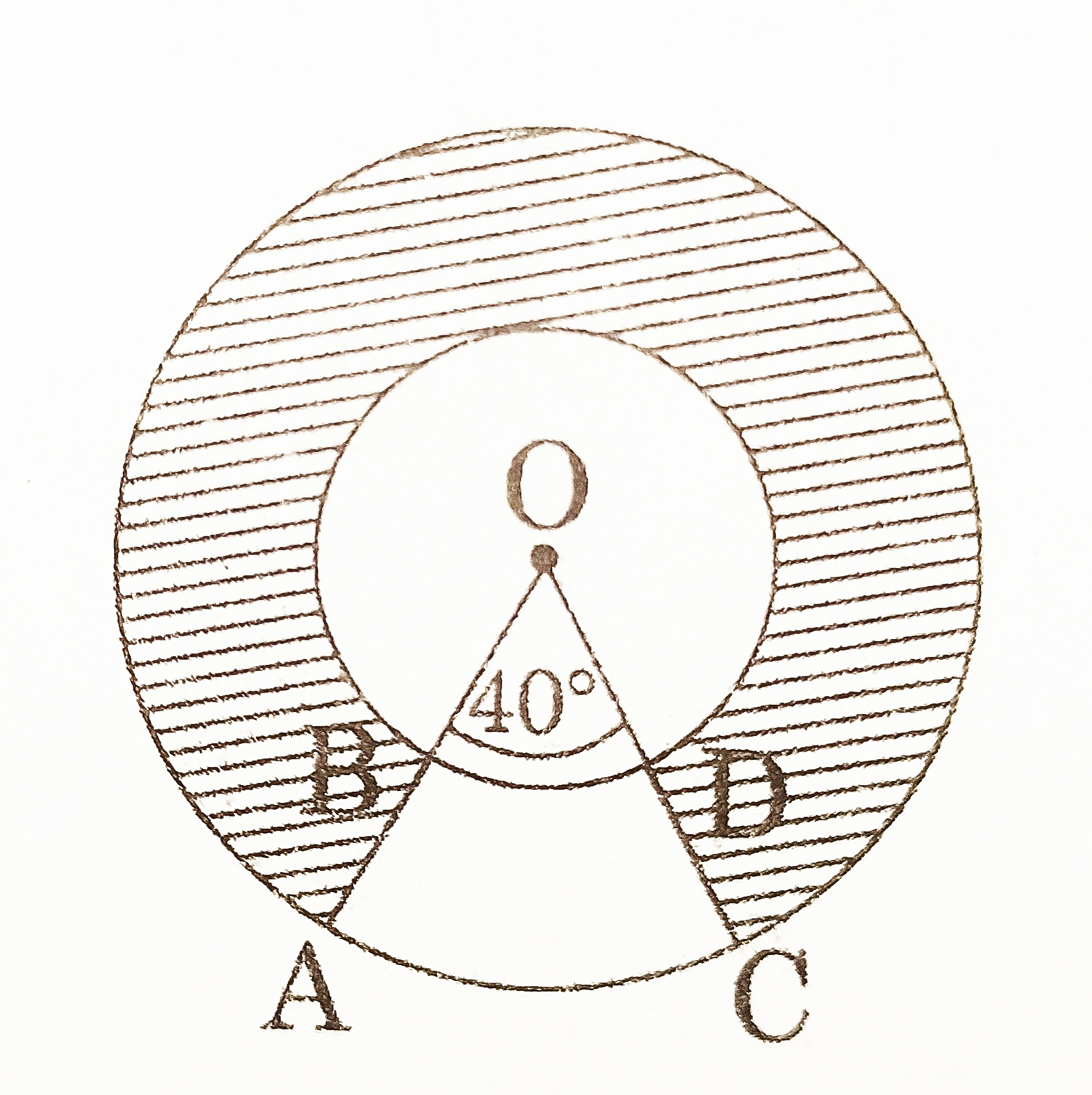[CBSE 2016] [3 Marks]
3. A momento is made as shown in the figure. Its base PBCR is silver plated from the front side. Find the area which is silver plated. (Use π = 22/7 )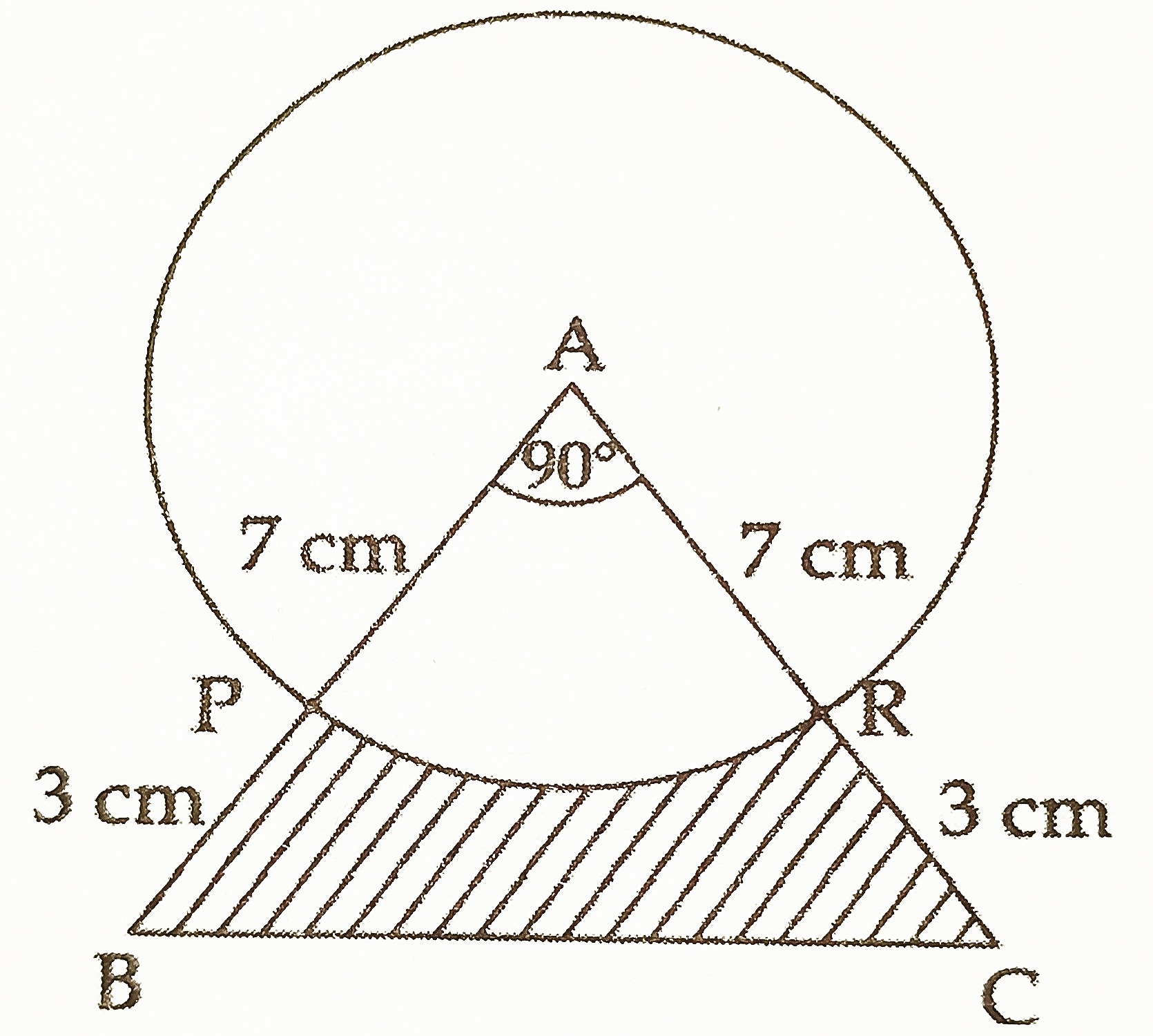[CBSE 2017] [3 Marks]
4. Find the area of the shaded region in figure, where arcs drawn with centres A, B, C and D intersect in pairs at mid point P, Q, R and S of the sides AB, BC, CD and DA respectively of a square ABCD of side 12 cm. (Take π = 3.14)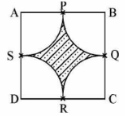[CBSE 2018] [3 Marks]
5. A chord of a circle of radius 14 cm subtends an angle of 60° at the centre. Find the area of the corresponding minor segment of the circle. (Use π = 22/7 and 3 = 1.73) [CBSE 2019] [3 Marks]
6. A car has two wipers which do not overlap. Each wiper has a blade of length 21 cm sweeping through an angle 120°. Find the total area cleaned at each sweep of the blades. (Use π = 22/7 ) [CBSE 2019] [3 Marks]
7. In the given figure, find the area of the shaded region where a circular arc of radius 7 cm has been drawn with vertex O of an equilateral triangle OAB of side 14 cm as centre. (Use π = 22/7 and 3 = 1.73 )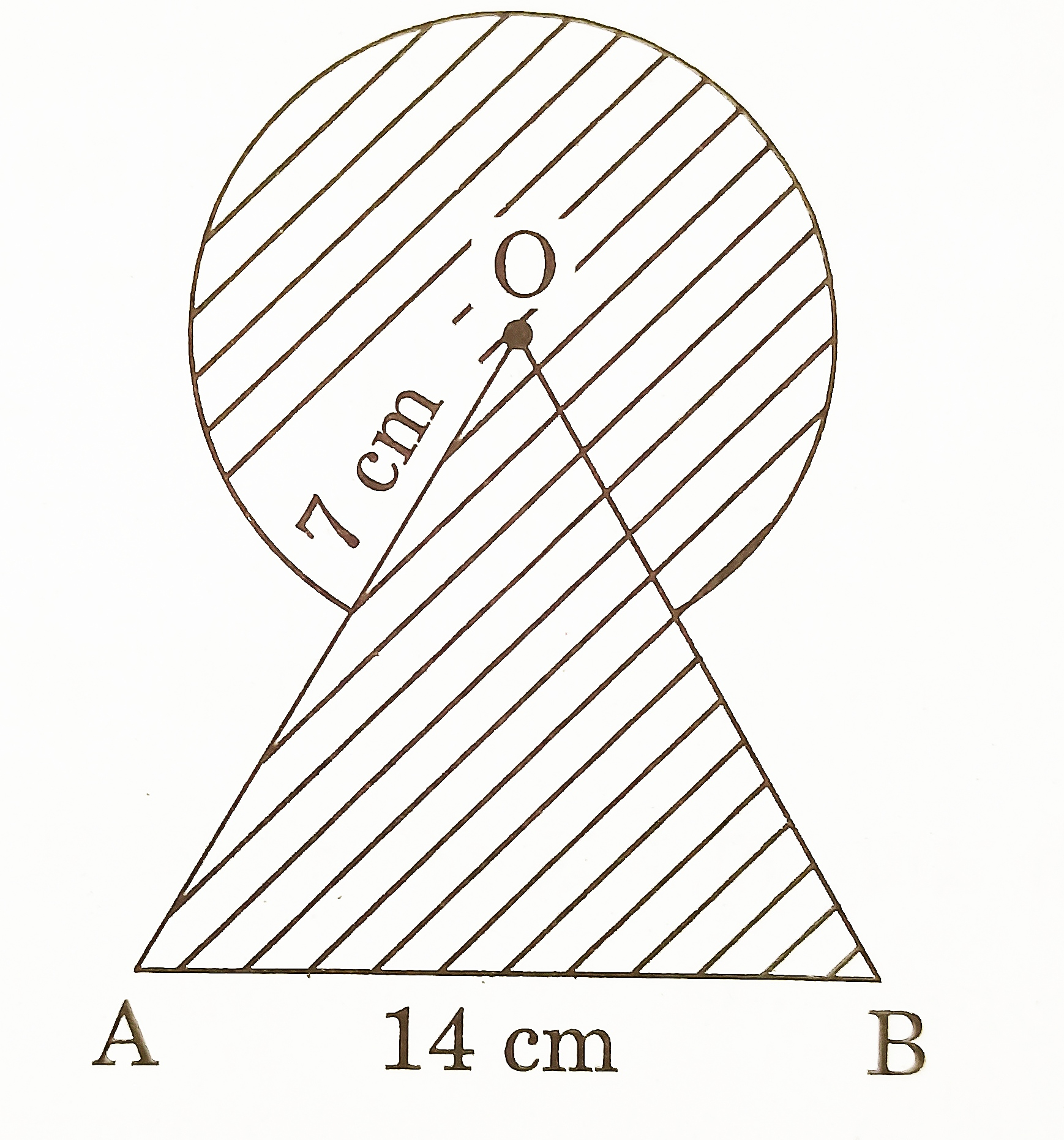[CBSE 2020] [3 Marks]
8. Calculate the area of the shaded region common between two quadrants of circles of radius 7 cm each (as shown in figure).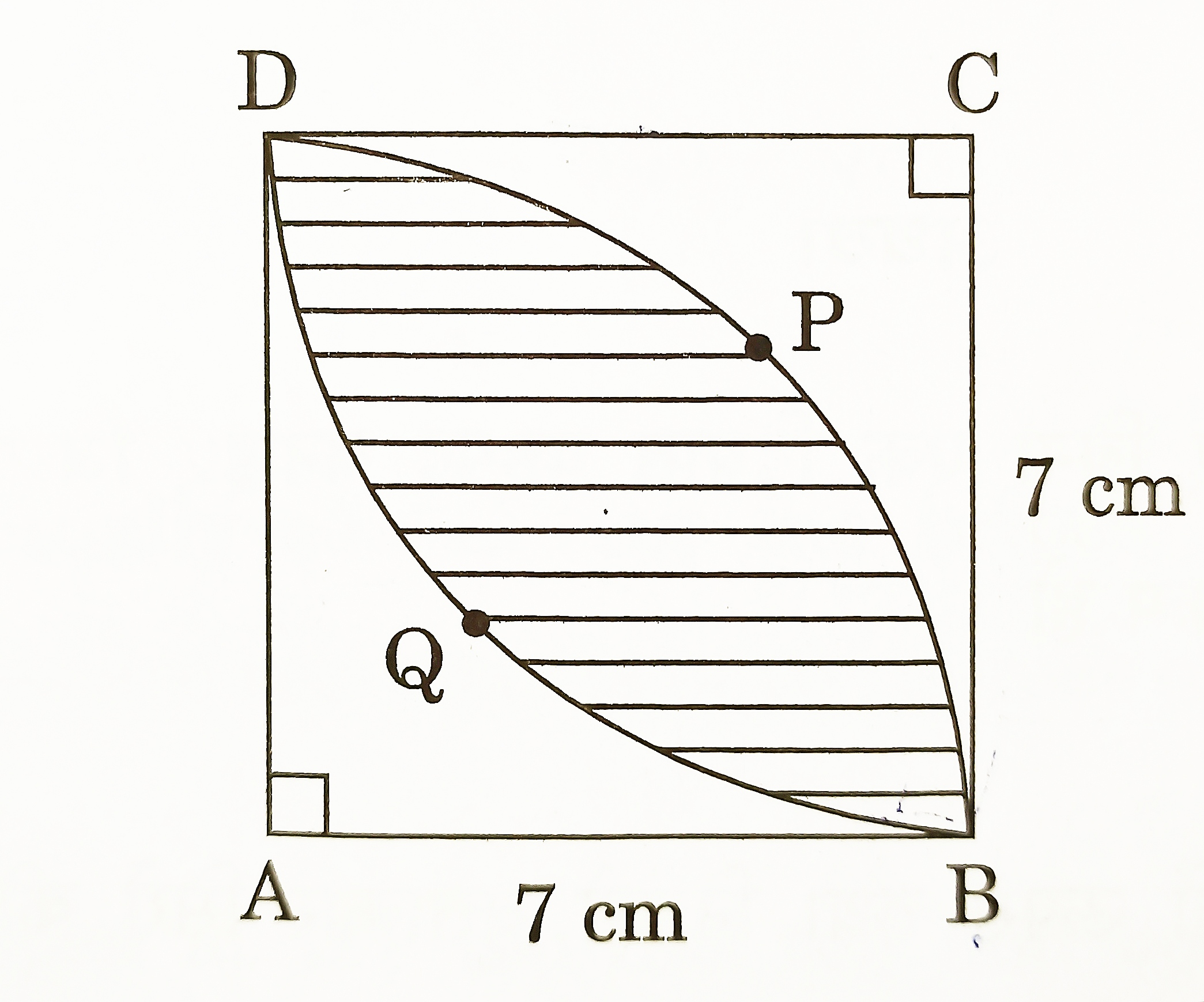[CBSE 2020] [3 Marks]
9. In the given figure, is shown a sector OAP of a circle with centre O, containing ∠θ. AB is perpendicular to the radius OA and meets OP produced at B. Prove that the perimeter of shaded region is r[ tan θ + sec θ + – 1 ].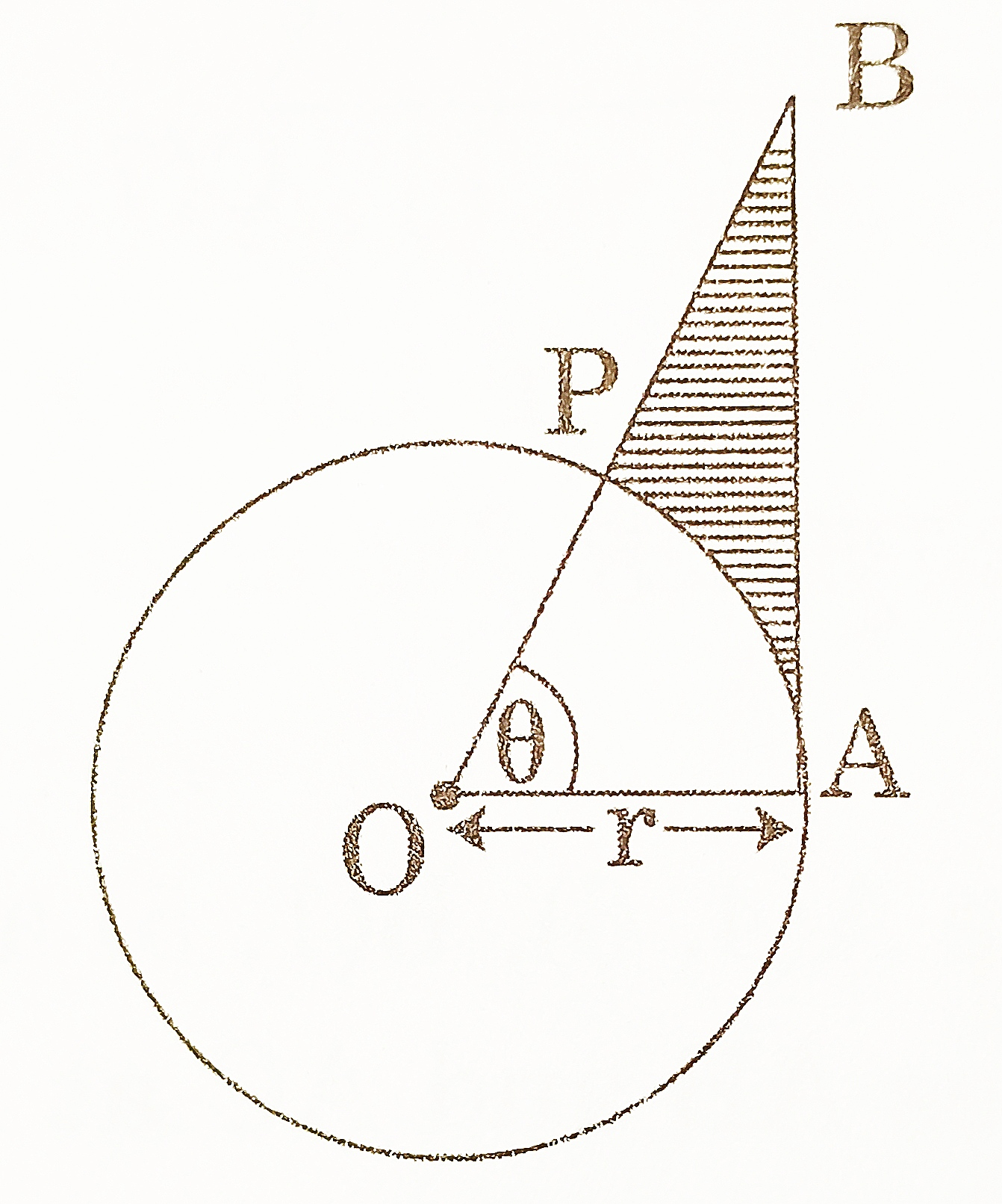[CBSE 2016] [4 Marks]